## # 队列

• 进行删除的一端称为队头。
• 进行插入的一端称为队尾。
• 先进先出原则：先入队的元素在队头，后入队的元素在队尾。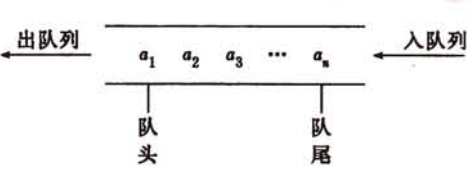• $a_1$ 称为队头元素；
• $a_n$ 称为队尾元素；
• 没有元素的队列称为空队列。

• 入队：插入数据到队尾。
• 出队：删除队头第一个元素。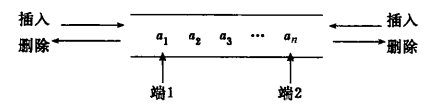• 输出受限的双端队列：一个端点只允许插入和删除，另一个端点只允许插入。
• 输入受限的双端队列：一个端点只允许插入和删除，另一个端点只允许删除。
• 双端队列也可以退化成栈，即只能在一端进行插入和删除。

• 向队尾添加元素(入队)
• 删除队首元素(出队)
• 获取队首的元素值(存取)
• 判断队列是否为满
• 判断队列是否为空

## # 顺序队列

• 元素出队后，只需将下一个元素设置成新队头。
优点：队列中其他元素地址无需改变；
缺点：随着元素不断出队，队头位置后移，可能出现存储空间不足的情况，从而导致新元素无法入队；同时队头前端存在大量空闲存储空间，造成空间的浪费。
• 队头元素必须存放在存储空间的第一个位置，元素出队后，所有元素向前移动一个位置。
优点：有效的利用了存储空间； 缺点：所有元素存储位置都要改变，时间效率低。

• $front$ 为队头指针，指示队头元素在顺序队列中的位置；
• $rear$ 为队尾指针，指示队尾元素的下一个位置，初始化时指向队头：$front == rear$
• $maxSize$ 表示队列的存储空间大小；
• $front == rear$ 时队空，当 $front == maxSize$ 时队列溢出，不能再添加元素。

#### # 顺序队列的初始化

• 申请容量为 $maxSize$ 的存储空间，$front$ 指向空间的基地址；
• $front == rear$

#### # 顺序队列的入队

• 判断队列是否溢出，若溢出不能添加元素；
• 否则将新元素入队，队尾指针加 1：$rear = rear + 1$

#### # 顺序队列之出队

• 判断队列是否为空，若队空则不能删除元素；
• 否则队头元素出队，队头指针加 1：$front = front + 1$

## # 循环队列

• 元素出队：队头指针顺时针移动一个位置；
• 元素入队：队尾指针顺时针移动一个位置。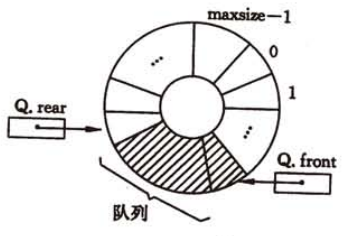• 新增变量 $count$ 表示队列元素个数，当 $count == maxSize$ 时队满；当 $count = 0$ 时队空。
• 循环队列少用一个元素，当队头指针在队尾指针的下一个位置时，队列为满，队列元素为 $maxSize - 1$ 个；当 $front == rear$ 时队空。
为了操作的便捷性，通常采用方式 2 判断队空队满，以下循环队列的操作以方式 2 为基准。

• 队满：$( rear + 1 ) \% maxSize == front$
• 队空：$front == rear$
• 队列中有效元素个数：$(rear + maxSize - front) \% maxSize$

#### # 循环队列的初始化

• 申请容量为 $maxSize$ 的存储空间，$front$ 指向空间的基地址。
• $front == rear$

#### # 循环队列的入队

• 判断循环队列是否队满，若队满则不能添加新元素
• 否则将新元素入队，队尾指针加 1：$rear = (rear + 1) \% maxSize$

#### # 循环队列之出队

• 判断队列是否为空，若队空则不能再删除元素
• 否则队头元素出队，队头指针加 1：$front = (front + 1) \% maxSize$

## # 链式队列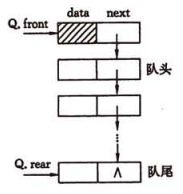• 队头指针：$front$ 始终指向头结点
• 队尾指针：$rear$ 指向队尾结点，初始化时 $front == rear$ 指向头结点

#### # 链式队列的初始化

• 创建一个新结点作为链式队列的头结点，并将队头和队尾指针指向头结点
• 头结点的指针域设为 $null$

#### # 链式队列的入队

• 创建指针域为 $null$ 的新结点 $x$
• 将新结点插入到队尾结点之后，修改队尾结点指针域：$rear.next = x$
• 修改队尾指针：$rear = x$

#### # 链式队列之出队

• 判断队列是否为空，若为空则不能删除结点
• 否则获取首元结点 $x$ 的数据域
• 删除首元结点，队头指针指向下一个结点：$front.next = x.next$
• 元素出队后再次判断队列是否为空，若为空将队尾指针指向头结点：$rear = front$
• 释放结点 $x$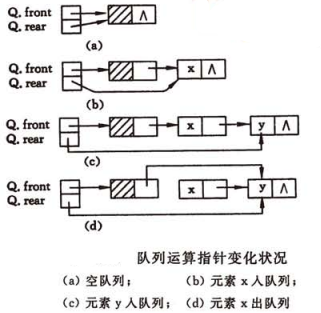#### # 循环队列和链式队列的比较

• 循环队列初始化时必须申请存储空间，队列的有效长度受限，在操作过程中队列的有效元素个数小于存储空间大小，造成空间浪费。
• 链式队列存储过程中需要一个指针域，会产生空间上的开销，但队列元素个数不会受限制。

• 银行拿号排队问题

## # 算法例题

1.滑动窗口最大值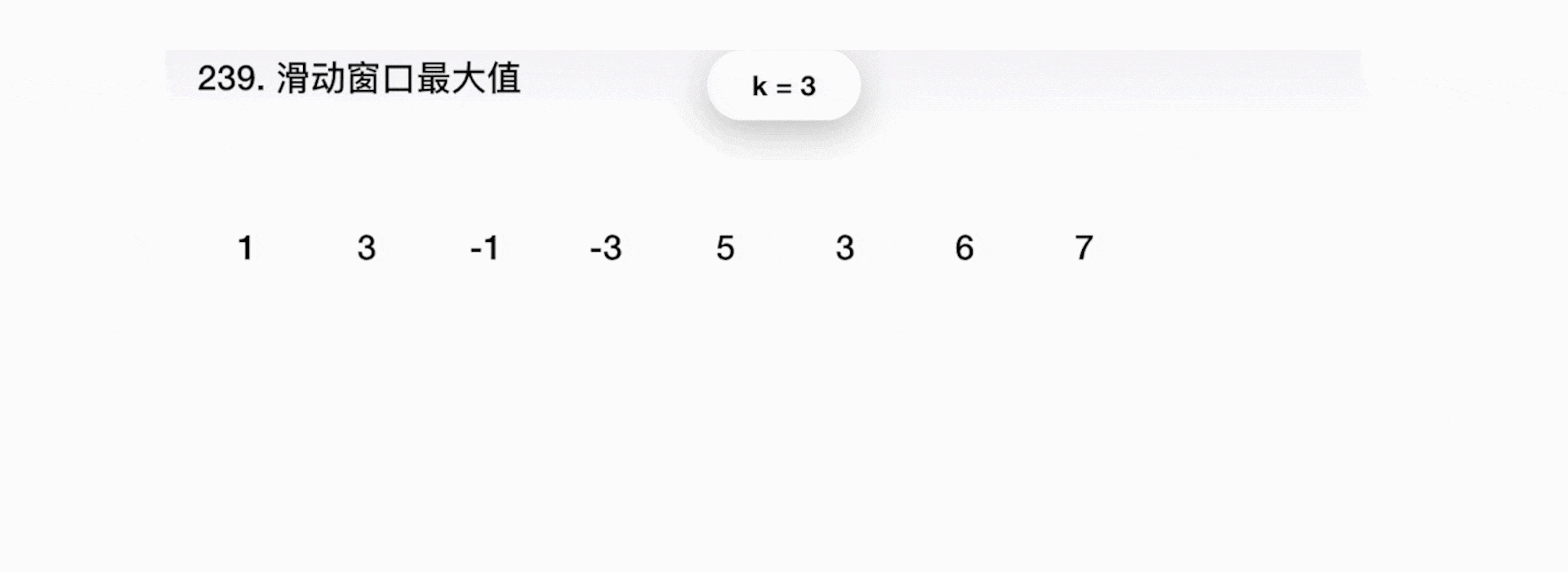1. 初始化窗口 k=3，包含 1，3，-1，把 1 的下标压入双端队列的尾部；
2. 把 3 和双端队列的队尾的数据逐个比较，3 >1，把 1 的下标弹出，把 3 的下标压入队尾；
3. -1<3，-1 压入双端队列队尾保留到下一窗口进行比较；
4. 3 为当前窗口的最大值；
5. 窗口移动，-3 与队尾数据逐个比较，-3<-1，-3 压入双端队列队尾保留；
6. 3 为当前窗口的最大值；
7. 窗口继续移动，5>-3，-3 从双端队列队尾弹出；
8. 5>-1，-1 从队尾弹出；
9. 3 超出当前窗口，从队列头部弹出；
10. 5 压入队列头部，成为当前窗口最大值；
11. 继续移动窗口，操作与上述同理。
窗口最大值只需读取双端队列头部元素。

## # 参考

• 《数据结构(C语言版)》 严魏敏、吴伟民著
• 《数据结构(第3版)》 刘大有等著
• 《搞定数据结构与算法》 苏勇